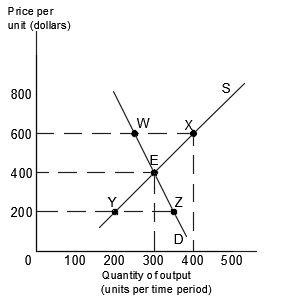# Refer to the above diagram. There is a surplus of about 100 units when the market price is A....

## Question:Refer to the above diagram. There is a surplus of about 100 units when the market price is

A. $800. B.$600.

C. $400. D. None of the above ## Surplus: In the market, we look at the consumers demand and producers supply. The point where both curve cut each other determines the quantity and price in the market. However, if the price is above the equilibrium price, there is a surplus in the market as demand is lesser at the higher price but producers are willing to supply much more that what is being demanded due to the good's higher price. ## Answer and Explanation: 1 Become a Study.com member to unlock this answer! Option b,$600 is the correct choice.

This is because we can clearly see in the above diagram that the equilibrium output is 300 units and the...